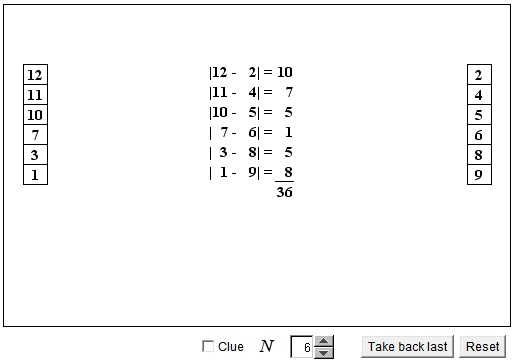# Proizvolov's Identity

At the beginning, the applet displays $2N$ natural numbers: $1, 2, 3, \ldots, 2N - 1, 2N.$ Select any $N$ numbers by clicking on $N$ numbers in turn. The selected numbers will be arranged in a column on the left in the decreasing order. The remaining $N$ numbers will be arranged in the increasing order on the right.

### Please try switching to IE 11 (Windows) or Safari (Mac), for no other browser nowadays runs Java applets. If asked whether to allow the applet to load, click Yes - the applet is signed with a security certificate from a trusted company.What if applet does not run?

Thus the set of numbers $\mathbb{N}_{2N} = \{1, 2, 3, \ldots, 2N - 1, 2N\}$ is split into two sequences, one decreasing and one increasing:

$a_{1} \gt a_{2} \gt a_{3} \gt \ldots \gt a_{N}$ and
$b_{1} \lt b_{2} \lt b_{3} \lt \ldots \lt b_{N}.$

The applet is supposed to suggest a theorem due to V. Proizvolov, a well known Russian mathematician:

$|a_{1} - b_{1}| + |a_{2} - b_{2}| + \ldots + |a_{N} - b_{N}| = N^{2}.$

The proof is based on the observation that

For every $i,$ $i = 1, \ldots, N,$ one of the numbers in the pair $\{a_{i}, b_{i}\}$ belongs to $\mathbb{N}_{N} = \{1, 2, \ldots, N\},$ the other to $\mathbb{N}_{N+1, 2N} = \{N+1, N+2, \ldots, 2N\}.$

### Proof 1

Assume on the contrary, that, for example, for some $i,$ both numbers in the pair belong to $\mathbb{N}_{N}:$

(1)

\begin{align} a_{i} &\lt N + 1\\ b_{i} &\lt N + 1. \end{align}

This would imply that (together with $a_{i})$ there are at the very least $(N - i + 1)$ terms of the sequence $\{a\}$ that are below $N + 1.$ There also would be at least $i$ terms from the second sequence $\{b\}$ below $N + 1.$ Together this would give $N + 1$ distinct natural numbers less than $N + 1.$ But there are only $N$ of them; thus our assumption (1) led to a contradiction.

The case

(2)

\begin{align} a_{i} &\gt N\\ b_{i} &\gt N. \end{align}

leads to a contradiction in a similar manner. Thus indeed, in any pair $a_{i}, b_{i}$ one number belongs to $\mathbb{N}_{N},$ the other to $\mathbb{N}_{N+1, 2N}.$ This implies that

\begin{align} |a_{1} - b_{1}| &+ |a_{2} - b_{2}| + \ldots + |a_{N} - b_{N}|\\ &= [(N+1) + (N+2) + \ldots + 2N] - [1 + 2 + \ldots + N]\\ &= [1 + 2 + \ldots + 2N] - 2·[1 + 2 + \ldots + N]\\ &= N(2N + 1) - N(N + 1)\\ &= N^{2}. \end{align}

### Proof 2

Let's assign the numbers in $\mathbb{N}_{N}$ one color, and another to the remaining numbers. It's a consequence of a general result that, if there is one monochrome pair, there necessarily is another one but of a different color. This leads to an immediate contradiction with the monotonicity of the sequences in two columns. Say, let one pair be $\{a',a''\},$ the other $\{b',b''\}.$ Then either $a'\lt b'$ and $a''\lt b''$ or $a'\gt b'$ and $a''\gt b'',$ a contradiction in both cases. From here the solution proceeds as before.

(This solution arose in an answer to a question posted by James Tanton on twitter.)

### Proof 3

There is an elegant geometric proof by Staurt Anderson. It can be found on a separate page.

### Proof 4

Grégoire Nicollier has observed that situation exploited by Proizvolov's identity can be generalized:

Let $S=\{a_1,\dots,\,a_n\}\cup\{b_1,\dots,\,b_n\}=\{c_1,\dots,\,c_{2n}\}$ be the given set of different numbers with $a_1\lt a_2\lt \cdots\lt a_n,$ $b_1\gt b_2\gt \cdots\gt b_n,$ $c_1\lt c_2\lt \cdots\lt c_{2n}.$ Then the sum

$\displaystyle\sum_{k=1}^{n}|a_{k}-b_{k}|$

is independent of the split of $S$ into the two so ordered sets.

Indeed, observe, that there is no pair $(a_k,\,b_k)$ whose both elements are smaller than $c_{n+1}$ or greater than $c_n$. Otherwise, there would be $k+(n+1-k)=n+1$ numbers in $S$ smaller than $c_{n+1}$ or greater than $c_n$, a contradiction. Each term $|a_k-b_k|$ is thus equal to $c_m-c_\ell$ for different $m$, $\ell$ with $\ell\le n\lt m$.

As an example, if $c_{k}=k^{2},$ then $\displaystyle\sum_{k=1}^{n}|a_{k}-b_{k}|=n^{2}(2n+1),$ independent of a particulare split of $S$ into two sets.

Grégoire also observed that the statement of the generalization holds when the inequalities are not required to be strict.

### References

1. S. Savchev, T. Andreescu, Mathematical Miniatures, MAA, 2003, p. 66.• Six integers out of 10: Pigeonhole Principle
• Pigeonhole Principle (Same sum)
• Pigeonhole in a Matrix
• Pigeonhole in Calendar
• A nice puzzle modeled on the Petersen graph
• Pigeonhole with Disjoint Intervals
• All antichains
• Euclid via Pigeonhole
• Light Bulbs in a Circle (an Interactive Gizmo)
• Seven integers under 127 and their Ratios
•